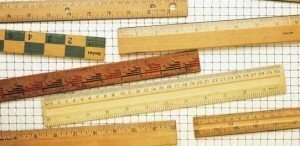# Measurement

There are many types of measurement tables and systems,but there are a few that have become standard. Note that this measurement guide gives only approximate figures.

### Cooking Measurement: Volume Equivalents

1 tsp is equal to 5 ml while 1 tbsp (1/2 fl oz) is the same as 15 ml. 1/4 cup (2 fl oz) is equal to 60 ml, while 1/3 cup is the same as 80 ml. 1/2 cup (4 fl oz) is equivalent to 120 ml. 2/3 cup is the same as 160 ml and 3/4 cup (6 fl oz) is equal to 180 ml.

1 cup (8 fl oz) is equal to 240 ml and the 1 qt (32 fl oz) is the same as 950 ml. 1 qt + 3 tbsps is equal to 1 L and 1 gal (128 fl oz) is the same as 4 L.

### Cooking Measurement Guide: Weight Equivalent

1/4 oz is equal to 7 g in the metric system and 1/2 oz is equal to 15 g. 3/4 oz is the same as 20 g and 1 oz is equal to 30 g. The 8 oz (1/2 lb) weight is equal to 225 g, while 12 oz (3/4 lb) is equal to 340 g. The 16 oz (1 lb) is equal to 455 g. The 35 oz (2.2 lbs) is equal to 1 kg.

### Linear Equivalents

These figures are also sometimes used n cooking. 1/4 in is equal to 0.75 cm and 1/2 in is the same as 1.50 cm. 3/4 in is equal to 2.00 cm. The metric equivalent of 1 in is 2.50 cm and for 6 in it is 15.00 cm. For 12 in (1 ft) the metric equivalent is 30.00 cm.

### Measurement Guide: Temperature

0 F is equal to -18 C (this is freezing temperature). Water freezes at 32 F or 0 C. 98.6 is equal to 37, while 180 F (water simmers) is equal to 82 C. 212 F (water boils) is equal to 100 C. 250 F (low oven) is equal to 120 C while 350 F is equivalent to 175 C.

### Other Cooking Measurements

An envelope of unflavored gelatin is equal to 1 tbsp while 250 grams of whipping cream is equivalent to 1 cup. 1 cup of sifted all-purpose flour is equal to 1 cup + 2 tbsp of cake flour (sifted). A cup of sifted cake flour is equivalent to 1 cup – 2 tbsp of all-purpose flour.

### Measurement Guide: Lengths and Weights

An inch is about 2.54 centimeters; a foot is 30.48 centimeters and a yard is 0.91 meters. An ounce is about 28.35 grams and a pound is 0.45 kilograms. 1 ton is equal to 0.91 metric tons.

An acre is 0.40 hectare while a sq mi is equal to 2.60 square kilometers. A cu ft is equal to 0.028 cubic meters. A square yard is about 0.84 square meters. A cu yd is 0.76 cubic meters.

Even though numbers can never be exactly translated from one system to another, the figures in this measurement guide are good enough to be used in everyday applications.